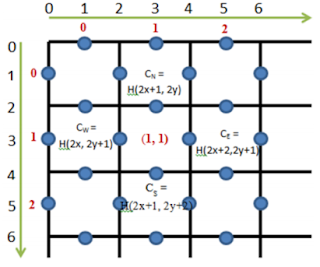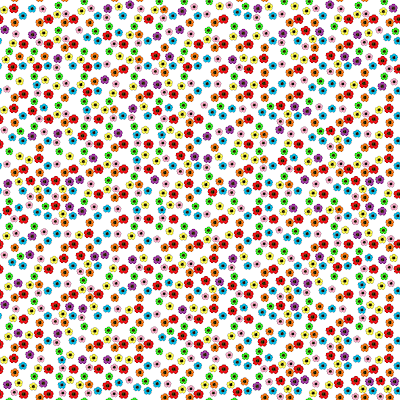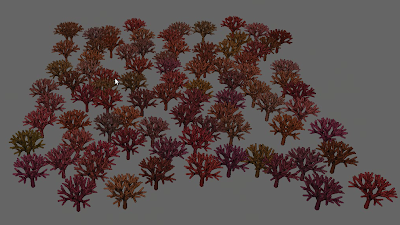## Introduction:

Repeating patterns is considered to be an issue in generating complex signals like textures and Poisson disk distribution. That problem is solved by Hao Wang, who proposed a method of non-periodic tiling using squared tiles with coloured edged.

This year, I did a Wang tiles related project that I really enjoyed. I decided to write about it in my blog and explain what Wang Tiles are and how Wang Tiles are used in Computer Graphics.

At the end of this post, I included a link with my demonstration video.

## Wang Tiles:

Wang Tiles are squared Tiles with coloured edges:Figure 1: A complete set of Wang Tiles

A plane is tiled with Wang Tiles using some randomness. But two edges can only be joined if they have the same colour.Figure 2: Image Generated by 40x40 Wang tiles

## Direct Stochastic Algorithm:

There are different algorithms of tiling a plane using a complete set of Wang Tiles.  The most efficient and quick is the Direct Stochastic Algorithm.

If the colours at the edges are known then the number of the tile can be calculated as follow:
T=CN*C^3+CW*C^2+CS^2+CE,
where:
T: the number of the tile,
C: the number of the colours and
CN, CW, CS, CE: the colours of the north, west, south and east edge accordingly

A double dense grid is used to calculate the colour of each edge, as shown below:Figure 3: Direct Stochastic Algorithm

The edge colours of the Wang Tile with coordinates  (x,y) is calculated as follow:
CN = hash(2x+1,2y),
CE = hash(2x+2,2y+1),
CS = hash(2X+1,2y+2),
CW = hash(2x,2y+1),
where the function hash(x,y) is a hash function that takes the coordinates of the tile and returns a number that represents a colour.

The code for the above algorithm is the following:

## Corner Tiles:

It was observed that sometimes unwanted artefacts appear at the corners of the Wang tiles. In 2006, Lagae and Dutre proposed corner’s based tiling method to solve that problem.

The following images are a complete set of Corner Tiles generated:Figure 4: A complete Set of Corner Tiles

Corner tiles are similar to Wang Tiles, but the corners are coloured instead of the edges.Figure 5: Image Generated using 40x40 Corner Tiles

The algorithm for tiling a plane using a set of Corner Tiles is easier, but creating the squared tiles is more difficult.

## Image/Texture Synthesis

It is an application area of Wang tiles in Computer Graphics. I have generated the following set of Tiles:Figure 6: complete set of Wang Tiles with flowers

and using my Wang Tiles related application I can create arbitrary large images with flowers:Figure 7: Image Synthesis

More output examples are shown in my demonstration video. Wang Tiles can be also used in shading languages, like Renderman, for procedural texturing.

The class diagram is very simple:

## Forest Generator:

Another application of Wang Tiles is the generation of Poison Disk Distribution, which can be used for anti-alising, Global illumination and Non-Photorealistic Rendering.

Creating Poisson disk Distributions is time expensive because its time complexity is relative to the amount of points. Using a pre-computed set of Wang Tiles, well distributed points can be generated in linear time.

For the Forest Generator, I created a complete set of Wang Tiles with points. The garden generator takes as input those Wang Tiles point sets and creates arbitrary large numbers of points that are well distributed in the space. Then a tree is drawn at each point and no collision between the trees exists.

Two output examples are shown below:Figure 9: Forest Generated using 3x3 Wang TilesFigure 10: Forest Generated using 9x9 Wang Tiles

## Demonstration Video:

The following video is a demonstration video of my application:

1.